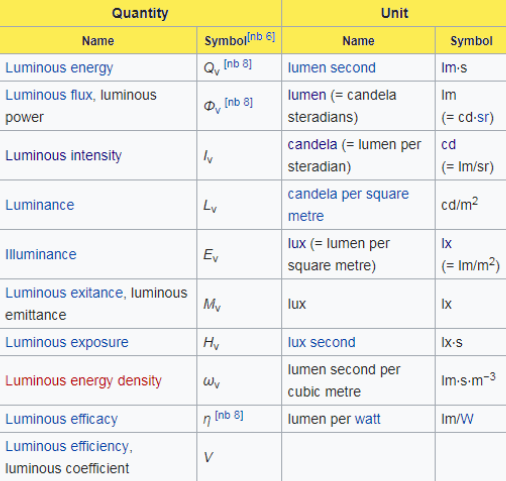Checkout JEE MAINS 2022 Question Paper Analysis : Checkout JEE MAINS 2022 Question Paper Analysis :

# Unit of Light

Well, when we talk about the unit of light it does not make much sense practically. It’s mainly because the light is not a physical quantity and as a result, it cannot be measured. However, light does have various physical properties like wavelength, intensity, speed, etc and these all can be measured. If you ask why? Well, the measurement helps in getting information mainly about the light wave or particle.

Since light has many properties and therefore, there are many different units for measuring them. While it can get quite complicated, let’s look at some of the few measurement units below.

## Candela (cd)

This SI unit is used to measure the luminous intensity of a light source which is moving in a specific direction. It is also sometimes referred to as a candle and represented as cd / m 2.

### Lumen (lm)

Another measurement unit, the lumen is actually a derived SI unit of luminous flux. It is represented by the symbol “lm” and is basically used to measure the output of artificial lights.

## Lux (lx)

Lux is basically the unit of illumination and is equal to one lumen per square metre. One lux equals 0.0929 footcandles and this is the metric equivalent of foot-candles. It is also known as a metre-candle. Notably, SI prefixes can also be used where one kilolux (klx) is equal to 1000 lux.

## Foot Candle (fc or ftc)

Light intensity can also be measured in terms of footcandle which is equivalent to lumens per square foot. In simple terms, this can be defined as the brightness of one candle covering a distance of one foot. One foot candle is approximately 10.7639 lux.

## Light Units TableRelated articles:

Test your knowledge on Unit Of Light